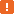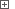# Obtaining Selected Records in Microsoft DataGridViewWhen testing your application with the DataGridView control, you may need to determine which records are currently selected in the grid in order to process them in a special way. You can obtain the selected rows, columns and cells currently selected using the grid’s `SelectedRows`, `SelectedColumns` and `SelectedCells` properties, respectively.

To obtain a particular item by its zero-based index within the returned collection, you can use the `Item(Index)` property. The total number of elements in the collection is specified by the `Count` property. However, in the case of large selections, using the `Count` property can significantly slow down the test performance. To improve the test performance, follow these tips:

• To determine whether all grid cells are selected, use the `grid.AreAllCellsSelected(True)` method.
• To check whether all cells currently visible in the grid are selected, use the `grid.AreAllCellsSelected(False)` method.
• To determine the total number of selected cells, use the `grid.GetCellCount("Selected")` method instead of the `grid.SelectedCells.Count` property.
• To determine the number of selected rows, use the `grid.Rows.GetRowCount("Selected")` method instead of the `grid.SelectedRows.Count` property.
• To determine the number of selected columns, use the `grid.Columns.GetColumnCount("Selected")` method instead of the `grid.SelectedColumns.Count` property.In order for TestComplete to have access to internal objects, properties and methods of the DataGridView control, the .NET Application Support and Microsoft Control Support plugins must be installed and enabled.

The following example demonstrates how you can obtain and process the records selected in the DataGridView control. It posts indices of the selected rows to the test log.

###### ExampleView description

JavaScript, JScript

function Main ()
{
var p, Grid, RowIndexes, i;

// Obtain the grid object
p = Sys.Process ("DataGridViewSample");
Grid = p.WinFormsObject("Form1").WinFormsObject("dataGridView1");

// Select a range of rows
SelectRowRange (Grid, 1, 6);

// Get the selected rows' indexes
RowIndexes = GetSelectedRowIndexes (Grid);

// Post the selected rows' cell values to the log
for (i=0; i<RowIndexes.length; i++)
Log.Message (RowIndexes[i] + ": " + Grid.wValue(RowIndexes[i], "Customer Name").ToString().OleValue);
}

// Returns an array of the selected rows' indexes
function GetSelectedRowIndexes (Grid)
{
var SelectedRows, nRows, i, arr;

// Get the collection of selected rows
SelectedRows = Grid.SelectedRows;

// Save the selected rows' indexes to an array
nRows = Grid.Rows.GetRowCount ("Selected");
arr = new Array (nRows);
for (i=0; i<nRows; i++)
arr[i] = SelectedRows.Item(i).Index;

return arr;
}

// Selects a range of rows
function SelectRowRange (Grid, StartRowIndex, EndRowIndex)
{
// Select the 1st row of the range
Grid.ClickRowIndicator (StartRowIndex);
// Extend the selection
Grid.ClickRowIndicator (EndRowIndex, skShift);
}

Python

``````def Main ():

# Obtain the grid object
p = Sys.Process ("DataGridViewSample");
Grid = p.WinFormsObject("Form1").WinFormsObject("dataGridView1");

# Select a range of rows
SelectRowRange (Grid, 1, 6);

# Get the selected rows' indexes
RowIndexes = GetSelectedRowIndexes (Grid);

# Post the selected rows' cell values to the log
for i in range(0, RowIndexes.length-1):
Log.Message (RowIndexes[i] + ": " + Grid.wValue[RowIndexes[i], "Customer Name"].ToString().OleValue);

# Returns an array of the selected rows' indexes
def GetSelectedRowIndexes (Grid):

# Get the collection of selected rows
SelectedRows = Grid.SelectedRows;

# Save the selected rows' indexes to an array
nRows = Grid.Rows.GetRowCount ("Selected");
arr = list(nRows);
for i in range(0, nRows-1):
arr[i] = SelectedRows.Item[i].Index;

return arr

# Selects a range of rows
def SelectRowRange (Grid, StartRowIndex, EndRowIndex):
# Select the 1st row of the range
Grid.ClickRowIndicator (StartRowIndex);
# Extend the selection
Grid.ClickRowIndicator (EndRowIndex, skShift);``````

VBScript

Sub Main
Dim p, Grid, RowIndexes, i

' Obtain the grid object
Set p = Sys.Process ("DataGridViewSample")
Set Grid = p.WinFormsObject("Form1").WinFormsObject("dataGridView1")

' Select a range of rows
Call SelectRowRange (Grid, 1, 6)

' Get the selected rows' indexes
RowIndexes = GetSelectedRowIndexes (Grid)

' Post the selected rows' cell values to the log
For i = 0 To UBound(RowIndexes)
Call Log.Message (CStr(RowIndexes(i)) & ": " & Grid.wValue(RowIndexes(i), "Customer Name").ToString().OleValue)
Next
End Sub

' Returns an array of the selected rows' indexes
Function GetSelectedRowIndexes (Grid)
Dim SelectedRows, nRows, i, arr

' Get the collection of selected rows
Set SelectedRows = Grid.SelectedRows

' Save the selected rows' indexes to an array
nRows = Grid.Rows.GetRowCount ("Selected")
arr = CreateVariantArray (0, nRows-1)
For i = 0 To nRows-1
arr(i) = SelectedRows.Item(i).Index
Next

GetSelectedRowIndexes = arr
End Function

' Selects a range of rows
Sub SelectRowRange (Grid, StartRowIndex, EndRowIndex)
' Select the 1st row of the range
Call Grid.ClickRowIndicator (StartRowIndex)
' Extend the selection
Call Grid.ClickRowIndicator (EndRowIndex, skShift)
End Sub

DelphiScript

function GetSelectedRowIndexes (Grid); forward;
procedure SelectRowRange (Grid, StartRowIndex, EndRowIndex); forward;

procedure Main;
var p, Grid, RowIndexes, i : OleVariant;
begin
// Obtain the grid object
p := Sys.Process ('DataGridViewSample');
Grid := p.WinFormsObject('Form1').WinFormsObject('dataGridView1');

// Select a range of rows
SelectRowRange (Grid, 1, 6);

// Get the selected rows' indexes
RowIndexes := GetSelectedRowIndexes (Grid);

// Post the selected rows' cell values to the log
for i:=0 to VarArrayHighBound(RowIndexes, 1) do
Log.Message (aqConvert.VarToStr(RowIndexes[i]) + ': ' + Grid.wValue[RowIndexes[i], 'Customer Name'].ToString().OleValue);
end;

// Returns an array of the selected rows' indexes
function GetSelectedRowIndexes (Grid);
var SelectedRows, nRows, i, arr : OleVariant;
begin
// Get the collection of selected rows
SelectedRows := Grid.SelectedRows;

// Save the selected rows' indexes to an array
nRows := Grid.Rows.GetRowCount ('Selected');
arr := CreateVariantArray (0, nRows-1);
for i := 0 to nRows-1 do
arr[i] := SelectedRows.Item[i].Index;

Result := arr;
end;

// Selects a range of rows
procedure SelectRowRange (Grid, StartRowIndex, EndRowIndex);
begin
// Select the 1st row of the range
Grid.ClickRowIndicator (StartRowIndex);
// Extend the selection
Grid.ClickRowIndicator (EndRowIndex, skShift);
end;

C++Script, C#Script

function Main ()
{
var p, Grid, RowIndexes, i;

// Obtain the grid object
p = Sys["Process"]("DataGridViewSample");
Grid = p["WinFormsObject"]("Form1")["WinFormsObject"]("dataGridView1");

// Select a range of rows
SelectRowRange (Grid, 1, 6);

// Get the selected rows' indexes
RowIndexes = GetSelectedRowIndexes (Grid);

// Post the selected rows' cell values to the log
for (i=0; i<RowIndexes["length"]; i++)
Log["Message"](RowIndexes[i] + ": " + Grid["wValue"](RowIndexes[i], "Customer Name")["ToString"]()["OleValue"]);
}

// Returns an array of the selected rows' indexes
function GetSelectedRowIndexes (Grid)
{
var SelectedRows, nRows, i, arr;

// Get the collection of selected rows
SelectedRows = Grid["SelectedRows"];

// Save the selected rows' indexes to an array
nRows = Grid["Rows"]["GetRowCount"]("Selected");
arr = new Array (nRows);
for (i=0; i<nRows; i++)
arr[i] = SelectedRows["Item"](i)["Index"];

return arr;
}

// Selects a range of rows
function SelectRowRange (Grid, StartRowIndex, EndRowIndex)
{
// Select the 1st row of the range
Grid["ClickRowIndicator"](StartRowIndex);
// Extend the selection
Grid["ClickRowIndicator"](EndRowIndex, skShift);
}